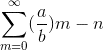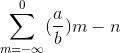# Summation Problem

baby_1
Hello
i want to know if we have a series with this equation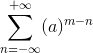and change it to this form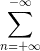the statement in Summation change or not?(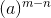this changes it right?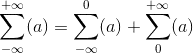where can i find the Summation notes?

(Assuming convergence) The first change makes no difference. The second change, you have n=0 twice.

baby_1
Thanks mathman
could you tell me how can i improve myself Summation? where can i find the Summation tips?

baby_1
Thanks mathman
i have another questions

you assume that series is: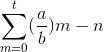can i multiple (-) (t and 0) ?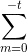if yes what about argument (it changed?)?

baby_1